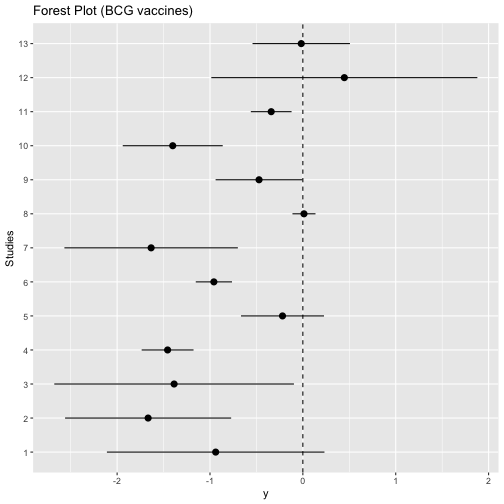## Introduction

To fit meta-analysis models using frequentist methods, there are many R packages available including `metafor`. On the other hand, Bayesian estimation methods such as Markov chain Monte Carlo (MCMC) are very attractive for meta-analysis, especially because they can be used to fit more complicated models. These include binomial-normal hierarchical models and beta-binomial models which are based on the exact distributional assumptions unlike (commonly used) normal-normal hierarchical model. Another advantage of Bayesian methods to be able to use informative prior distributions for example to regularize heterogeneity estimates in case of low number of studies. Thus, we developed `MetaStan` which uses Stan (a modern MCMC engine) to fit several pairwise meta-analysis models including binomial-normal hierarchical model and beta-binomial model. This package is also the accompanying package for Günhan, Röver, and Friede (2020).

## Installation

The development version of `MetaStan` is available on Github (https://github.com/gunhanb/MetaStan) and can be installed using `devtools` package as follows:

``````devtools:::install_github("gunhanb/MetaStan")
``````

The BCG trials example is available in the package, and it can be loaded as follows:

``````library("MetaStan")
data("dat.Berkey1995", package = "MetaStan")
#   Trial  rt    nt  rc    nc Latitude
# 1     1   4   123  11   139       44
# 2     2   6   306  29   303       55
# 3     3   3   231  11   220       42
# 4     4  62 13598 248 12867       52
# 5     5  33  5069  47  5808       13
# 6     6 180  1541 372  1451       44
``````

Additional information can be obtained by typing `?dat.Berkey1995` (for any dataset and function in the package).

We can visualize individual log odds ratio estimates plot using `ggplot2` as follows:

``````library(ggplot2)
# Calculating log odds ratios and variances from data
logodds <- function(x) log((x * (x - x))/((x - x) * x))
stdes   <- function(x) sqrt(1/x + 1/(x - x) + 1/x + 1/(x - x))
r_ind   <- apply(cbind(dat.Berkey1995\$rt, dat.Berkey1995\$nt,
dat.Berkey1995\$rc, dat.Berkey1995\$nc), 1, logodds)
se_ind  <- apply(cbind(dat.Berkey1995\$rt, dat.Berkey1995\$nt,
dat.Berkey1995\$rc, dat.Berkey1995\$nc), 1, stdes)
lower95_ind <- r_ind + qnorm(.025) * se_ind
upper95_ind <- r_ind + qnorm(.975) * se_ind
# Comparison of the results
trials  <- c("1", "2" ,"3", "4", "5", "6", "7", "8", "9", "10", "11", "12", "13")
trials <- ordered(trials, levels = trials)

d <- data.frame(x = trials,
y = r_ind,
ylo = lower95_ind,
yhi = upper95_ind)
forest.plot <- ggplot(d, aes(x = x, y = y, ymin = ylo, ymax = yhi)) +
geom_pointrange() +
coord_flip() +
geom_hline(aes(yintercept=0), lty = 2) +
xlab("Studies") +
ggtitle("Forest Plot (BCG vaccines)")

plot(forest.plot)
``````## Fitting a binomial-normal hierarchical model

`metastan` is the main fitting function of this package. The main computations are executed via the `rstan` package's `sampling` function. We can fit the binomial-normal hierarchical model (Günhan, Röver, and Friede, 2020) using a weakly informative prior for treatment effect as follows:

``````bnhm1.BCG.stan  <- meta_stan(ntrt = nt,
nctrl = nc,
rtrt = rt,
rctrl = rc,
data = dat.Berkey1995,
tau_prior_dist = "half-normal",
tau_prior = 0.5,
theta_prior = c(0, 2.82),
model = "BNHM1",
chains = 4,
iter = 2000,
warmup = 1000)
``````

Convergence diagnostics, very conveniently, obtained using `shinystan` package as follows:

``````library("shinystan")
## Firstly convert "stan" object to a "shinystan" object
bnhm1.BCG.shinystan = as.shinystan(bnhm1.BCG.stan\$fit)
launch_shinystan(bnhm1.BCG.shinystan)
``````

A simple summary of the fitted model is given by `print` option:

``````print(bnhm1.BCG.stan)
# Meta-analysis using MetaStan
# Mean treatment effect
#  mean  2.5%   50% 97.5%
# -0.75 -1.15 -0.75 -0.37
# Heterogeneity stdev
#  mean  2.5%   50% 97.5%
#  0.59  0.36  0.57  0.93
``````

Note that this model corresponds to Model 4 in Jackson et al (2018). The model 2 in Jackson et al (2018) can be fitted by specfying `model = "BNHM2"` as follows:

``````bnhm2.BCG.stan  <- meta_stan(ntrt = nt,
nctrl = nc,
rtrt = rt,
rctrl = rc,
data = dat.Berkey1995,
theta_prior = c(0, 2.82),
tau_prior_dist = "half-normal",
tau_prior = 0.5,
model = "BNHM2")
``````
``````print(bnhm2.BCG.stan)
# Meta-analysis using MetaStan
# Mean treatment effect
#  mean  2.5%   50% 97.5%
# -0.76 -1.15 -0.75 -0.39
# Heterogeneity stdev
#  mean  2.5%   50% 97.5%
#  0.60  0.36  0.58  0.95
``````

Please see Günhan, Röver, and Friede (2020) and Jackson et al (2018) for complete model descriptions.

# References

Günhan, BK, Röver, C, and Friede, T (2020). “Random-effects meta-analysis of few studies involving rare events”. In: Research Synthesis Methods 11.1, pp. 74-90. DOI: 10.1002/jrsm.1370.

Jackson, D et al. (2018). “A comparison of seven random-effects models for metaanalyses that estimate the summary odds ratio”. In: Statistics in Medicine 37.7, pp. 1059-1085. DOI: 10.1002/sim.7588.